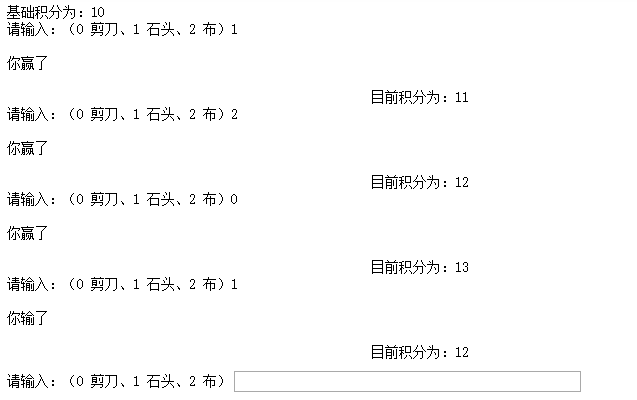• 任务要求在控制台中提示输入石头、剪刀、布，按回车键，然后给出游戏结果。...那就该用到python中的一个模块random中的一个方法random.randint()在0~2范围内产生一个随机整数，就表电脑出拳了。random.rand...
任务要求
在控制台中提示输入石头、剪刀、布，按回车键，然后给出游戏结果。
分析
我们知道在游戏规则中，石头克剪刀，剪刀克布，布克石头。但是这在计算机中并不是很好直接的表示，因此我们分别用0、1、2分别代表游戏中的石头剪刀布。
那么电脑该如何出拳呢？那就该用到python中的一个模块random中的一个方法random.randint()在0~2范围内产生一个随机整数，就表电脑出拳了。
random.randint()的用法如下：
# 首先导入模块
import random
# 调用时传入两个整型数字，返回从1-2中任意一个整型数字
a = random.randint(1,2)
# 打印出1或者2
print(a)
# 再或者,那么b就可能是1-10中的任意一个数字（包括1和10）
b = random.randint(1,10)
print(b)
#在本案例中，我们只要0-2中的任意一个数即可，故
computer = random.randint(0,2)
玩家出拳的话，可以从键盘输入0-2中的任意一个数并保存在一个变量中，然后用if语句比较得出结果即可。
代码实现：
import random
# 从键盘获取用户的输入,只能输入0-2中的数字，否则结果会不正确，以后会有升级版
person = input('请输入：石头(0)、剪刀(1)、布(2)：')
# input 返回的是一个字符串类型，randint(0, 2)返回的是int类型，需要把person强制转换成int类型，类型一致才可以比较
person = int(person)
computer = random.randint(0, 2)
# 为了更友好的显示信息
if person == 0:
print('玩家：石头')
elif person == 1:
print('玩家：剪刀')
else:
print('玩家：布')
if computer == 0:
print('电脑：石头')
elif computer == 1:
print('电脑：剪刀')
else:
print('电脑：布')
# 如果出拳一样就是平局
if person == computer:
print('你好厉害呀！居然和我打成平局！')
# 玩家：石头 电脑：剪刀
# 玩家：剪刀 电脑：布
# 玩家：布 电脑：石头 这三种情况下玩家赢
elif person == 0 and computer == 1 or person == 1 and computer == 2 or person == 2 and computer == 0:
print('恭喜你，你赢了！')
# 其他情况都是玩家输
else:
print('真遗憾，你输了！')
运行示意图
​以上就是本文的全部内容，希望对大家的学习有所帮助，也希望大家多多支持我们。
时间： 2019-08-27
展开全文• python猜拳游戏电脑随机循环版 import random while True: # 1.让用户输入 player = int(input("请出拳 石头(1)剪刀(2)布(3):")) # 2.计算机产生随机数 computer = random.randint(1, 3) # 3.比较胜负 if(...
python猜拳游戏电脑随机循环版
import random
while True:
# 1.让用户输入
player = int(input("请出拳 石头(1)剪刀(2)布(3):"))
# 2.计算机产生随机数
computer = random.randint(1, 3)
# 3.比较胜负
if((player == 1) and (computer == 2)) or ((player ==2 ) and (computer == 3)) or ((player ==3)and(computer == 1)):
print("玩家胜利")
elif player == computer:
print("平局")
else:
print("电脑胜利")

展开全文• python开发猜拳游戏的逻辑代码，简单实用有效，python新学者需要的下载看看
• ## python猜拳

千次阅读 2019-06-17 15:39:51
用random模块使电脑随机出拳，然后用while True一直循环下去 import random # 导入随机模块 sum=10 # 积分 print("基础积分为：10") # ...
用random模块使电脑随机出拳，然后用while True一直循环下去
import random                                      # 导入随机模块
sum=10                                             # 积分
print("基础积分为：10")                            # 显示基础积分
A=("剪刀","石头","布")
while True:
a=input("请输入：（0 剪刀、1 石头、2 布）")   # 将输入的数据转化为整型
c=random.randint(0,2)                         # 随机一个0到2的整数
if a.isdigit() and a in ['0','1','2']:        # 是否为数字,且在出拳数字内
a=int(a)                                  # 转换为整型
if a==c:                                  # 平局条件
print("\n平局\n")
elif a-c==1 or c-a==2:                    # 赢局条件
print("\n你赢了\n")
sum+=1
else:                                     # 输局
print("\n你输了\n")
sum-=1
print("你出%s，电脑出%s" % (A[a], A[c]))          # 显示出双方出拳
else:                                         # 输入错误
print("你没正确出拳")
print(("目前积分为：%d"%sum).rjust(60))展开全文• //判断玩家出拳，则进入相对应的循环 //注: dianNao = 0为石头， dianNao = 1为剪刀， dianNao = 2为布 电脑会根据自己的随机数判断输赢，输入错误则跳过此循环并进行下次当前回合循环 if(wangJia=="石头"): if...
//导入随机数

import random;

//输入的回合总数

huiHeSum = int(input("请输入回合数:"))

//当前的回合数

thisHuiHe = 0;

print();

print("----------对战开始----------");

//电脑得分

dianNaoNum = 0;

//玩家得分

wangJiaNum = 0;

//先让玩家一直出拳

while(True):

//创建一个石头剪刀布的数组

quan = ["石头","剪刀","布"];

//让电脑随机一个(0~2)的数去对应数组的下标

dianNao = random.randint(0,2);

print();

print("第{}回合".format(thisHuiHe+1 ));

//玩家出拳回合

wangJia = input("你的回合--请出拳:");

//电脑出拳回合

print("电脑回合--请出拳:"+quan[dianNao]);

//判断玩家输入是否正确

if(wangJia=="石头" or wangJia=="剪刀" or wangJia=="布"):

//输入正确当前回合加一

thisHuiHe = thisHuiHe +1;

//平局判断

if quan[dianNao]==wangJia:

print("平局");

//判断玩家出拳，则进入相对应的循环

//注: dianNao = 0为石头，

dianNao = 1为剪刀，

dianNao = 2为布

电脑会根据自己的随机数判断输赢，输入错误则跳过此循环并进行下次当前回合循环

if(wangJia=="石头"):

if(dianNao==1):

print("玩家胜");

wangJiaNum = wangJiaNum+1;

elif(dianNao==2):

print("电脑胜");

dianNaoNum = dianNaoNum+1;

elif(wangJia=="剪刀"):

if(dianNao==0):

print("电脑胜");

dianNaoNum = dianNaoNum+1;

elif(dianNao==2):

print("玩家胜");

wangJiaNum = wangJiaNum+1;

elif(wangJia=="布"):

if(dianNao==0):

print("玩家胜");

wangJiaNum = wangJiaNum+1;

elif(dianNao==1):

print("电脑胜");

dianNaoNum = dianNaoNum+1;

else:

print("出拳错误!无法判定");

continue;

//如果当前回合数=输入的回合总数，则游戏结束并打印对 战结果，然后程序结束

if(thisHuiHe ==huiHeSum ):

print();

print("----------游戏结束!对战结果----------");

print("对战回合:",huiHeSum );

print("电脑得分:",dianNaoNum);

print("玩家得分:",wangJiaNum);

if(dianNaoNum>wangJiaNum):

print("最终结果:","电脑胜");

elif(dianNaoNum<wangJiaNum):

print("最终结果:","玩家胜");

elif(dianNaoNum==wangJiaNum):

print("最终结果:","平局");

break;

执行结果:

请输入回合数:5

----------对战开始----------

第1回合

你的回合--请出拳:剪刀

电脑回合--请出拳:石头

电脑胜

第2回合

你的回合--请出拳:石头

电脑回合--请出拳:石头

平局

第3回合

你的回合--请出拳:布

电脑回合--请出拳:剪刀

电脑胜

第4回合

你的回合--请出拳:剪刀

电脑回合--请出拳:石头

电脑胜

第5回合

你的回合--请出拳:石头

电脑回合--请出拳:布

电脑胜

----------游戏结束!对战结果----------

对战回合: 5

电脑得分: 4

玩家得分: 0

最终结果: 电脑胜

展开全文• 原标题：用python实现“猜拳"游戏 用python实现“猜拳”游戏先来练习一道用python编写的小程序这道题是用for in 循环实现输入10个数并求和。这里用到了append()方法append( )方法:是一个很重要的方法，它是向列表的...
• 猜拳游戏 分析类：属性、方法 自己玩家：Player 选角色[“美羊羊”，“喜羊羊”,"沸羊羊"] ​ 出拳 石头 剪刀 布 计算机玩家：Computer 选角色[“1美羊羊”，“喜洋洋”,"沸羊羊"] 随机选角 ​ 随机出拳 game类，...
• while 1:#这是一个死循环 print('1.石头 2.剪刀 3.布') person = int(input('请出拳：')) computer = random.randint(1,3) #输出机器人的出拳结果 if computer==1: print('机器人出的是：石头') pass elif ...
• 首先，解释一下人机猜拳猜拳相信大家都了解，通俗讲就是“石头、剪刀、布”嘛！再简单不过的一个小游戏，而所谓人机猜拳其实就是猜拳的双方中一方是人另一方是计算机。问题一人的问题好解决，计算机怎么能随机出拳...
• Python,while循环小例子--猜拳游戏(三局二胜)import randomall_choice = ['石头', '剪刀', '布']prompt = '''(0)石头(1)剪刀(2)布请选择(0\1\2)'''# 人的计分板pwin = 0# 计算机的计分板cwin = 0# 人和计算机都没有...
• 首先，解释一下人机猜拳猜拳相信大家都了解，通俗讲就是“石头、剪刀、布”嘛！再简单不过的一个小游戏，而所谓人机猜拳其实就是猜拳的双方中一方是人另一方是计算机。问题一人的问题好解决，计算机怎么能随机出拳...
• ##循环   """ while 条件： 执行语句 打印1-100的数字 i=1 while i print(i) i+=1 """ 打印1-100的偶数： i=1 while i if i%2==0: print(i) i+=1 i = 0 while i ...
• #加上循环循环猜拳 while True: player=int(input("输出0（拳），1（剪），2（布）")) com_player=random.randint(0,2) #等于3时，退出游戏 if player==3: print("退出游戏~") break #退出循环 i...
• Python 编程中 while 语句用于循环执行程序，即在某条件下，循环执行某段程序，以处理需要重复处理的相同任务。其基本形式为：while 判断条件：执行语句……执行语句可以是单个语句或语句块。判断条件可以是任何...
• import random while True: computer = random.randint(1, 3) player = int(input("请输入您的状态代号，石头请输：1、剪子请输：2、布请输：3: ")) if ((player == 1) and (computer == 2)) or ((player == 2) ...python
• Python 编程中 while 语句用于循环执行程序，即在某条件下，循环执行某段程序，以处理需要重复处理的相同任务。其基本形式为：while判断条件：执行语句……执行语句可以是单个语句或语句块。判断条件可以是任何...
• python猜拳游戏的实现，不是很难，很容易看懂，也很容易理解
• 对于循环循环 标准数据类型  数字（数字）  String（字符串）  清单（列表）  元组（元组）  字典（字典）   数字：  用于存储数值。数据类型是不允许改变的，这就意味着如果改变数字数据类型得...
• 今天的这篇文章呢是对人机猜拳小游戏——石头剪刀布的一个描述以及代码展现石头剪刀布游戏代码的简介：关于石头剪刀布这个小游戏，大致得到思路就是，玩家出一个手势，然后电脑再随机出一个手势，最后再判断是玩家...
• 这篇文章主要介绍了Python While循环语句实例演示及原理解析,文中通过示例代码介绍的非常详细，对大家的学习或者工作具有一定的参考学习价值,需要的朋友可以参考下Python 编程中 while 语句用于循环执行程序，即在某...
• import random from time import sleep def inputquan(): global value value = input("你出啥啊？") if value == "剪刀": caiquan(0) elif value == "石头": caiquan(1) elif value =...
• 猜拳游戏 用户输入0到2任意一个数字，分别定义为石头、剪刀、布，与随机生成数进行猜拳。 #石头剪刀布游戏：石头：0，剪刀:1,布：2 #引入随机数库 import random #将输入内容强制转化为数字 a=int(input("请输入一...
• Python 编程中 while 语句用于循环执行程序，即在某条件下，循环执行某段程序，以处理需要重复处理的相同任务。其基本形式为： while 判断条件： 执行语句…… 执行语句可以是单个语句或语句块。判断条件可以是...
• [python]猜拳小游戏 知识点： 1、循环体 2、try，except 3、if，elif，else 4、random() import random print ("开始猜拳游戏！") print("你可以输入 0 退出游戏。") while True: try: b=int(input("石头：1，...
• Python 编程中 while 语句用于循环执行程序，即在某条件下，循环执行某段程序，以处理需要重复处理的相同任务。其基本形式为： while 判断条件： 执行语句…… 执行语句可以是单个语句或语句块。判断条件可以是...
• Python新手入门具体实例python中的循环运用（打印水仙花数，一到一百内猜拳） 1.用for，while循环来打印100-1000的水仙花数并进行统计 2.合理运用循环来进行六次1-100以内的猜数字 1.关于水仙花的例子 水仙花数是指...for while...

# python猜拳循环python 订阅